Courses

# Intermittency Notes | EduRev

## Civil Engineering (CE) : Intermittency Notes | EduRev

The document Intermittency Notes | EduRev is a part of the Civil Engineering (CE) Course Fluid Mechanics.
All you need of Civil Engineering (CE) at this link: Civil Engineering (CE)

Intermittency

• Consider a turbulent flow confined to a limited region. To be specific we shall consider the example of a wake (Figure 33.1a), but our discussion also applies to a jet (Figure 33.1b), a shear layer (Figure 33.1c), or the outer part of a boundary layer on a wall.

• The fluid outside the turbulent region is either in irrotational motion (as in the case of a wake or a boundary layer), or nearly static (as in the case of a jet). Observations show that the instantaneous interface between the turbulent and nonturbulent fluid is very sharp.

• The thickness of the interface must equal the size of the smallest scales in the flow, namely the Kolmogorov microscale.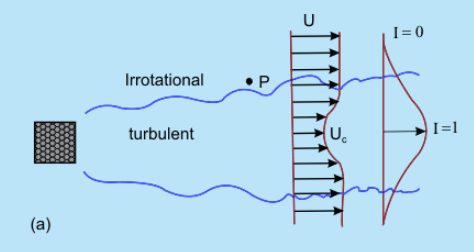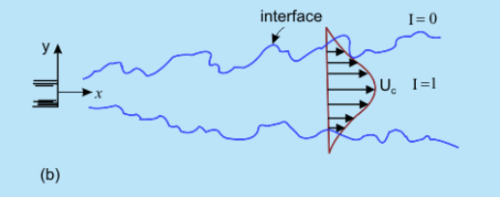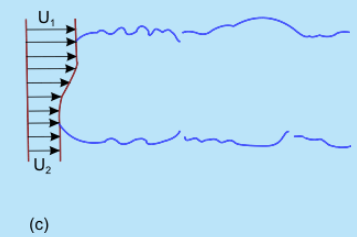Figure 33.1  Three types of free turbulent flows; (a) wake  (b) jet and (c) shear layer [after P.K. Kundu and I.M. Cohen, Fluid Mechanics, Academic Press, 2002]

• Measurement at a point in the outer part of the turbulent region (say at point P in Figure 33.1a) shows periods of high-frequency fluctuations as the point P moves into the turbulent flow and low-frequency periods as the point moves out of the turbulent region. Intermittency I is defined as the fraction of time the flow at a point is turbulent.

• The variation of I across a wake is sketched in Figure 33.1a, showing that I =1 near the center where the flow is always turbulent, and I = 0 at the outer edge of the flow domain.

Derivation of Governing Equations for Turbulent Flow

• For incompressible flows, the Navier-Stokes equations can be rearranged in the form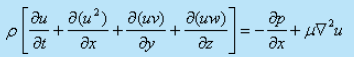(33.1a)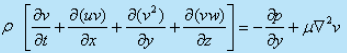(33.1b)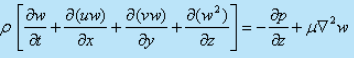(33.1c)

and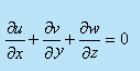(33.2)

• Express the velocity components and pressure in terms of time-mean values and corresponding fluctuations. In continuity equation, this substitution and subsequent time averaging will lead to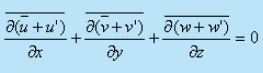or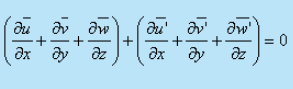since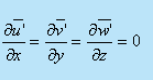We can writeFrom Eqs (33.3a) and (33.2), we obtain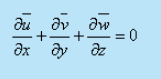(33.3a)

• It is evident that the time-averaged velocity components and the fluctuating velocity components, each satisfy the continuity equation for incompressible flow.
• Imagine a two-dimensional flow in which the turbulent components are independent of the -direction. Eventually, Eq.(33.3b) tends to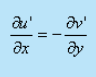On the basis of condition (33.4), it is postulated that if at an instant there is an increase in u' in the -direction, it will be followed by an increase in v' in the negative -direction. In other words,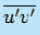is non-zero and negative. (see Figure 33.2)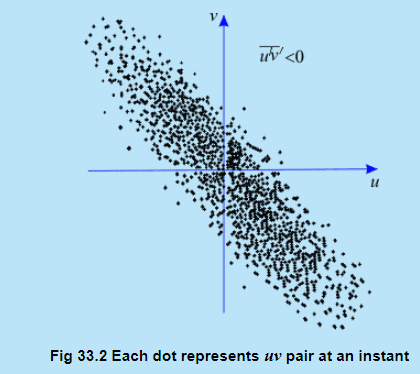Invoking the concepts of eqn. (32.8) into the equations of motion eqn (33.1 a, b, c), we obtain expressions in terms of mean and fluctuating components. Now, forming time averages and considering the rules of averaging we discern the following. The terms which are linear, such as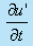and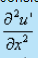vanish when they are averaged [from (32.6)]. The same is true for the mixed terms like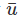,u' or, v but the quadratic terms in the fluctuating components remain in the equations. After averaging, they form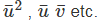Contd. from previous slide

If we perform the aforesaid exercise on the x-momentum equation, we obtain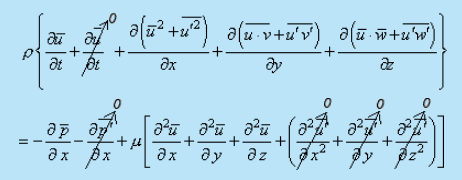using rules of time averages,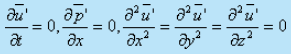We obtain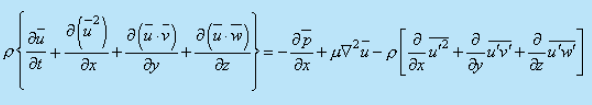Introducing simplifications arising out of continuity Eq. (33.3a), we shall obtain.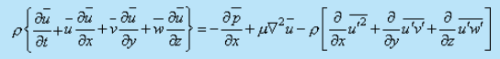• Performing a similar treatment on y and z momentum equations, finally we obtain the momentum equations in the form.

In x direction,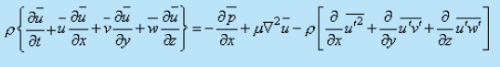(33.5a)

In y direction,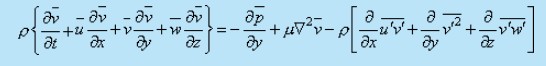In z direction,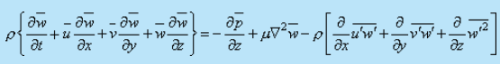• Comments on the governing equation :

1. The left hand side of Eqs (33.5a)-(33.5c) are essentially similar to the steady-state Navier-Stokes equations if the velocity components u,v and are replaced by,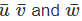2. The same argument holds good for the first two terms on the right hand side of Eqs (33.5a)-(33.5c).

3. However, the equations contain some additional terms which depend on turbulent fluctuations of the stream. These additional terms can be interpreted as components of a stress tensor.

• Now, the resultant surface force per unit area due to these terms may be considered as

In x direction,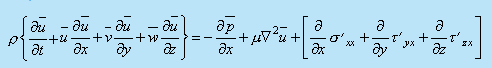(33.6a)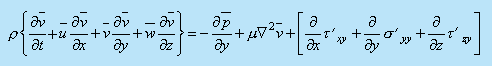(33.6b)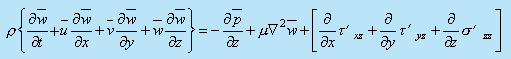(33.6c)

• Comparing Eqs (33.5) and (33.6), we can write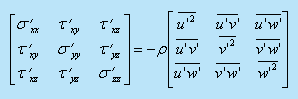(33.7)

• It can be said that the mean velocity components of turbulent flow satisfy the same Navier-Stokes equations of laminar flow. However, for the turbulent flow, the laminar stresses must be increased by additional stresses which are given by the stress tensor (33.7). These additional stresses are known as apparent stresses of turbulent flow or Reynolds stresses . Since turbulence is considered as eddying motion and the aforesaid additional stresses are added to the viscous stresses due to mean motion in order to explain the complete stress field, it is often said that the apparent stresses are caused by eddy viscosity . The total stresses are now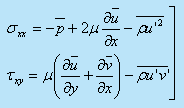(33.8)

and so on. The apparent stresses are much larger than the viscous components, and the viscous stresses can even be dropped in many actual calculations .

Turbulent Boundary Layer Equations

• For a two-dimensional flow (w = 0)over a flat plate, the thickness of turbulent boundary layer is assumed to be much smaller than the axial length and the order of magnitude analysis may be applied. As a consequence, the following inferences are drawn: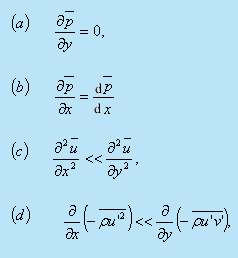The turbulent boundary layer equation together with the equation of continuity becomes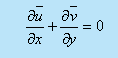(33.9)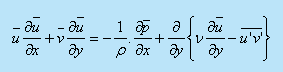(33.10)

• A comparison of Eq. (33.10) with laminar boundary layer Eq. (23.10) depicts that: u, v and p are replaced by the time average values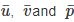,and laminar viscous force per unit volume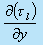is replaced by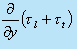where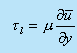is the laminar shear stress and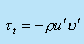is the turbulent shear stress.

Boundary Conditions

• All the components of apparent stresses vanish at the solid walls and only stresses which act near the wall are the viscous stresses of laminar flow. The boundary conditions, to be satisfied by the mean velocity components, are similar to laminar flow.
• A very thin layer next to the wall behaves like a near wall region of the laminar flow. This layer is known as laminar sublayer and its velocities are such that the viscous forces dominate over the inertia forces. No turbulence exists in it (see Fig. 33.3).
• For a developed turbulent flow over a flat plate, in the near wall region, inertial effects are insignificant, and we can write from Eq.33.10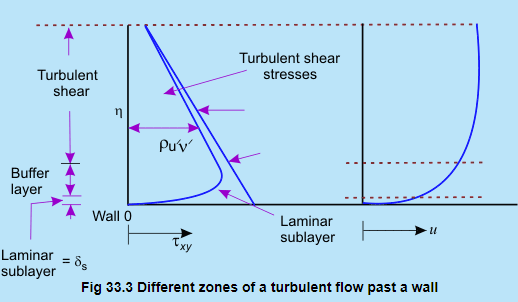which can be integrated as ,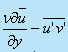=constant

• We know that the fluctuating components, do not exist near the wall, the shear stress on the wall is purely viscous and it follows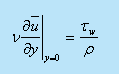However, the wall shear stress in the vicinity ofthe laminar sublayer is estimated as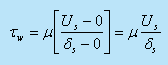( 33.11a)

where Us is the fluid velocity at the edge of the sublayer. The flow in the sublayer is specified by a velocity scale (characteristic of this region).

• We define the friction velocity,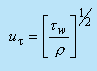as our velocity scale. Once ur is specified, the structure of the sub layer is specified. It has been confirmed experimentally that the turbulent intensity distributions are scaled with ur . For example, maximum value of the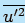is always about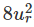. The relationship between ur and the Us can be determined from Eqs (33.11a) and (33.11b) as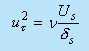Let us assume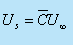Now we can write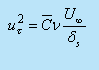where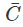is a proportionality constant          (33.12a)

or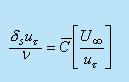(33.12b)

Hence, a non-dimensional coordinate may be defined as,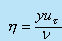which will help us estimating different zones in a turbulent flow. The thickness of laminar sublayer or viscous sublayer is considered to be n ≈ 5

Turbulent effect starts in the zone of n > 5 and in a zone of 5< n < 70, laminar and turbulent motions coexist. This domain is termed as buffer zone. Turbulent effects far outweight the laminar effect in the zone beyond n = 70 and this regime is termed as turbulent core .

• For flow over a flat plate, the turbulent shear stress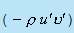is constant throughout in the y direction and this becomes equal to Tw at the wall. In the event of flow through a channel, the turbulent shear stressvaries with and it is possible to write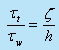(33.12c)

where the channel is assumed to have a height 2h and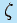is the distance measured from the centreline of the channel (= h - y). Figure 33.1 explains such variation of turbulent stress.

Shear Stress Models

In analogy with the coefficient of viscosity for laminar flow, J. Boussinesq introduced a mixing coefficient μr for the Reynolds stress term, defined as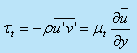Using μr the shearing stresses can be written as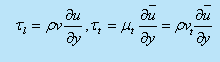such that the equation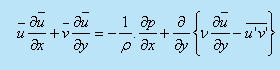may be written as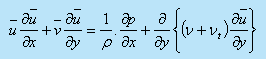(33.13)

The term vt is known as eddy viscosity and the model is known as eddy viscosity model

Unfortunately the value of v is not known. The term vt is a property of the fluid whereas νt is attributed to random fluctuations and is not a property of the fluid. However, it is necessary to find out empirical relations between vt, and the mean velocity. The following section discusses relation between the aforesaid apparent or eddy viscosity and the mean velocity components

Prandtl's Mixing Length Hypothesis

• Consider a fully developed turbulent boundary layer . The stream wise mean velocity varies only from streamline to streamline. The main flow direction is assumed parallel to the x-axis (Fig. 33.4).
• The time average components of velocity are given by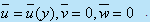. The fluctuating component of transverse velocity v' transports mass and momentum across a plane at y1 from the wall. The shear stress due to the fluctuation is given by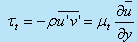• Fluid, which comes to the layer y1 from a layer (y- 1) has a positive value of v'. If the lump of fluid retains its original momentum then its velocity at its current location y1 is smaller than the velocity prevailing there. The difference in velocities is then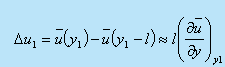(33.15)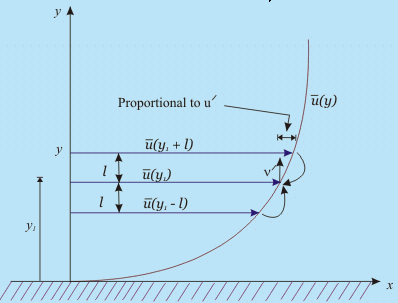Fig. 33.4   One-dimensional parallel flow and Prandtl's mixing length hypothesis

The above expression is obtained by expanding the function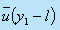in a Taylor series and neglecting all higher order terms and higher order derivatives. l is a small length scale known as Prandtl's mixing length . Prandtl proposed that the transverse displacement of any fluid particle is, on an average, 'l' .

continued..

Consider another lump of fluid with a negative value of v'. This is arriving at y1 from ( y+ 1). If this lump retains its original momentum, its mean velocity at the current lamina y1 will be somewhat more than the original mean velocity of y1. This difference is given by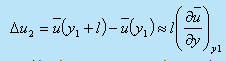(33.16)

• The velocity differences caused by the transverse motion can be regarded as the turbulent velocity components at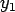• We calculate the time average of the absolute value of this fluctuation as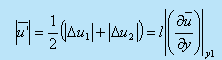(33.17)

• Suppose these two lumps of fluid meet at a layer y1 The lumps will collide with a velocity 2u' and diverge. This proposes the possible existence of transverse velocity component in both directions with respect to the layer at y1. Now, suppose that the two lumps move away in a reverse order from the layer y1 with a velocity 2u'. The empty space will be filled from the surrounding fluid creating transverse velocity components which will again collide at y1. Keeping in mind this argument and the physical explanation accompanying Eqs (33.4), we may state that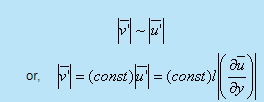along with the condition that the moment at which u' is positive, v' is more likely to be negative and conversely when u' is negative. Possibly, we can write at this stage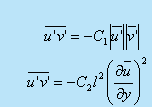(33.18)

where C1 and C2 are different proportionality constants. However, the constant C2 can now be included in still unknown mixing length and Eg. (33.18) may be rewritten as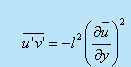• For the expression of turbulent shearing stress τt we may write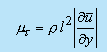• After comparing this expression with the eddy viscosity Eg. (33.14), we may arrive at a more precise definition,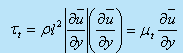(33.20a)where the apparent viscosity may be expressed as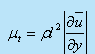(33.20b)and the apparent kinematic viscosity is given by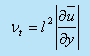(33.20c)

• The decision of expressing one of the velocity gradients of Eq. (33.19) in terms of its modulus as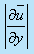was made in order to assign a sign to τt according to the sign of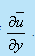.
• Note that the apparent viscosity and consequently,the mixing length are not properties of fluid. They are dependent on turbulent fluctuation.
• But how to determine the value of "l" the mixing length? Several correlations, using experimental results for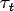have been proposed to determine l.

However, so far the most widely used value of mixing length in the regime of isotropic turbulence is given by

where Y is the distance from the wall and is known as von Karman constant  (≈ 0.4 ).

Offer running on EduRev: Apply code STAYHOME200 to get INR 200 off on our premium plan EduRev Infinity!

## Fluid Mechanics

43 videos|70 docs|72 tests

,

,

,

,

,

,

,

,

,

,

,

,

,

,

,

,

,

,

,

,

,

;